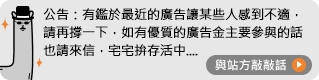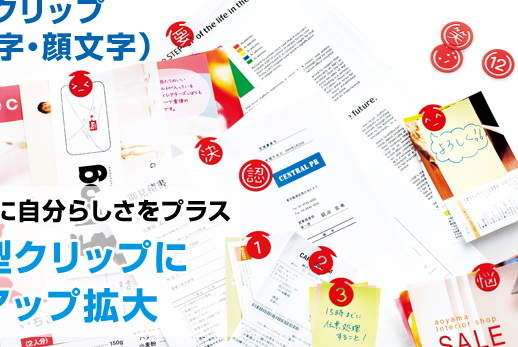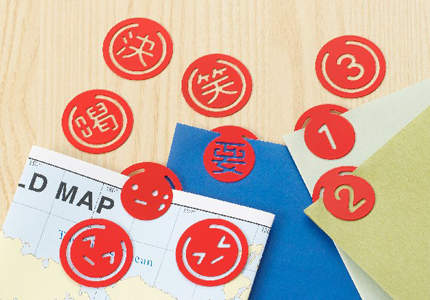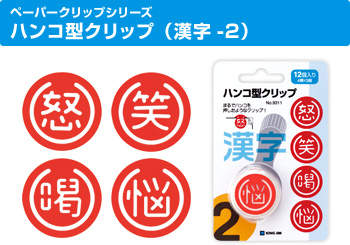# 上班文件小物：顏文字文件夾＆顏文字係蝦密### 文件夾有三種型式可以選擇「顏文字」這個詞的意思就是指用文字和符號組成表情或圖案來表達撰寫者的心情，

XD 笑翻

:-)  微笑。

:-(  不悅。

;-)  使眼色。

:-D  開心。

:-P  吐舌頭。

:-C  很悲傷。

:-O  驚訝, 張大口。

:-/  懷疑。

8-)  戴眼鏡者的微笑。

xc== 嘔。

--<-<-<@ 送你一朵玫瑰花。

ο-_-)０)。０)      給你一拳~~

m(_ _)m 萬事拜託(跪地，嗑頭)

(/_) 看不到~眼睛被手摭住了

|(- _-)| 沒聽到~耳朵遮住了

(⊙０⊙)  目瞪口呆

⊙ . ⊙ 睜大眼

(^0^)/ 舉手歡呼

－ －|||| 無奈

?~? 疑問、疑問

◎−◎？ 疑問

(T_T) 傷心、我哭了

ＱＱ 流淚

Ｑ０Ｑ 流淚、張大嘴

〒△〒 哭

T△T 哭訴.......

U／／／U 臉紅紅害羞 (小明是常用 >///< )

≧◇≦ 感動~

╮(－_－)╭ 兩手一攤~

＝　＝＃ 生氣（青筋暴露）

= 3 = 嘟嘴

( (－_－)y--~~ 抽煙......

(/‵Ⅲ′)/ 抓你來咬!!!

(Q o Q) b 人家才沒有

*(^_^)/* 拿彩球、為你加油~

(#‵′) 啍!!

(>_<) 小生氣

_ /# 我生氣了(横眉豎眼)

(*^．^*) 親一個!!!

(@^０^@)/★ 晚安~~

(>﹏<) 不!!!!!!!!!!!11

(*+﹏+*)~@ 受不了~受不了

‵(*∩_∩*)′ 獻上最可愛的笑容

(×_×) 昏倒

........(><)/ 哇!!出現了!!

=  =b 冒冷汗

^ ^" 笑笑的無奈

(－_－)ｚＺ 睡著了啦～

－▽－ｙ 耶！ ∪＿∪ 恩恩～

((。(^_^)。)) 期待、期待

<(‵▽′)> 哈哈哈~~(我是壞人~)

"(/><)/ 阿達~~ (>c<) 唉唉叫~

(^^;;;;; 緊張、緊張~~

(╯︵╰) 一臉苦瓜

p(^_^)q 加油~(兩手緊握拳高舉)

f(^_^) 這樣啊...

<("""○""")> Oh!  My God

(．Q．) 做鬼臉

(*^^)/.。．：*：．。★，。：*。☆ 祝好夢

("*_*)(‵~~‵)==== 看到鬼，就是看到鬼了嘛

(_ _)(- . -)(~0~) 累了~又瞇眼~又打呵欠的~

(^o^哇)~~(^0^)哈~~(^○^)哈~~ 大笑三聲~~

0(^o^)~~YA(^0^)~~SU(^○^)~~MI(^_^) 好好休息(用於睡前~日文)

～＞＿＜～　　　　　　　　哭泣中．．．

o_O  訝異

=_="  無奈

(￣﹁￣)   流口水

( h_n )   大眼睛和小眼睛

‧★,:*:‧(￣▽￣)/‧:*‧°★*   用力灑花恭喜

OrzＯ|￣|＿敗給你了（一個人五體投地的象形符號，有許多衍生變化，例：Orz  Or2

(-ิ_-ิ;)、(-ิo-ิ) 泰國式顏文字

＜（￣︶￣）＞　　　　　滿足且得意，得意快樂

加油!加油!加油!

＜（￣︶￣）／　　　　　　滿足並且伸出手，表示贊同、贊成GO!

＜（＠￣︶￣＠）＞　　　　滿足地臉紅

（＃￣▽￣＃）　　　　　　害羞

＼（￣︶￣）／　　　　　　抱抱，開心

ｂ（￣▽￣）ｄ　　　　　　豎起雙手拇指說好

╰（￣▽￣）╭　　　　　　眉飛色舞

{>_<}

（￣︶￣）↗　　　　　　　出發吧

○（＊￣︶￣＊）○　　　　吃飽睡好

♀（￣▽￣）／　　　　　　優質女孩

♂（￣▽￣）／　　　　　　優質男孩

╮（╯◇╰）╭　　　　　　啾～啾～親一個愛你>-<

（┬＿┬）　　　　　　　　流淚

（＞﹏＜）　　　　　　　　痛苦

（┬＿┬）↘　　　　　　　跌倒

〒▽〒　　　　　　　　　　真命苦

（︶︿︶）                不滿

╰（‵□′）╯            暴怒

（︶︿︶）＝凸            比中指

（╯－＿－）╯╧╧        翻桌A

（╯‵□′）╯︵┴─┴    翻桌B

╭∩╮（￣▽￣）╭∩╮    哼，去你的（鄙視你）

（～￣▽￣～） 裝傻

╭(─╴╴─)╮ ▃▇ 嗯嗯中，別打擾

o(〒﹏〒)o 淚

O-(///￣皿￣)☞ ─═≡☆゜★█▇▆▅▄▃▂＿ 佛地魔版之去去武器走

( ☉_☉)≡☞o────★° 哈利波特版之去去武器走

○(￣︿￣) ○─═★° 榮恩版之去去武器走

「囧」 是 代表 無可奈何的臉（原為「光明」、「明亮」的意思）

╮(￣▽￣")╭  無奈

ψ(._. )>      筆記A

≧ω≦ 可愛、可愛

O口O!　　　　　超驚訝!

、表情符號-雙人篇

（╬￣皿￣）＝○＃（￣＃）３￣）             看拳

＜（‵□′）＞───Ｃε（┬＿┬）３          生氣扭耳朵

＜( ￣︿￣)︵θ︵θ︵θ︵θ︵☆（＞口＜－）     無影腳攻擊

(((^ ^)(^ ^))) 什麼什麼

(⊙_⊙)<~~啾~~>(#^_^#) 親一個

(^ ^)／▽▽＼(^ ^) 乾杯~~杯子碰杯子

(^_^)／★＼(^_^) 乾杯(杯子相碰)

(^_-)db(-_^) 手指打勾勾~約定!

╭（′▽‵）╭（′▽‵）╭（′▽‵）╯　　　　GO!

（￣▽￣）～■□～（￣▽￣）　　　　　　　　　乾杯

＜（￣︶￣）＞＜（￣︶￣）＞＜（￣︶￣）＞　　當我們同在一起

、表情符號-非人類篇

<。)#)))≦        烤魚

(‧‧)mmm        毛毛蟲

(0^◇^0)/ 麻雀

<*)>>>=< 魚骨頭

(=^ ^=) 貓

/(*w*) 免子

^(00)^ 小豬

(：≡ 水母

(。。)~ 蝌蚪

<□：≡ 烏賊

@/" 蝸牛

Σ^)/ 烏鴨

ζ。≡ 獅子

(￣(工)￣) 大狗熊

--<-<-<@ 玫瑰花

(((●< 蟑螂

<※ 花束

### 不過不是只有我們人類會顏文字喔～,,,,,,,,,,,,,,,,,,,,,,,,,,,

>>> 點這裡看更多 就是愛閒聊 相關討論

0 則回應 順序: